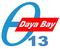TWiki > TWikiVariables > VarCALC Daya Bay webs: Public | 中文 | Internal | Help Log In or Register

### CALC{"formula"} -- add spreadsheet calculations to tables

• The `%CALC{formula}%` variable is handled by the SpreadSheetPlugin. Over 100 functions are available, such as `\$ABS()`, `\$EXACT()`, `\$EXISTS()`, `\$GET()/\$SET()`, `\$IF()`, `\$LOG()`, `\$LOWER()`, `\$PERCENTILE()`, `\$TIME()`, `\$VALUE()`.
• Syntax: `%CALC{formula}%`
• Examples:
• `%CALC{\$SUM(\$ABOVE())}%` returns the sum of all cells above the current cell
• `%CALC{\$EXISTS(Web.SomeTopic)}%` returns `1` if the topic exists
• `%CALC{\$UPPER(Collaboration)}%` returns `COLLABORATION`
• Note: The CALC variable is handled with delay compared to other TWiki variables. You may get unexpected results if you nest CALC inside other variables (such as `%INCLUDE{%CALC{...}%}%`) because it does not get evaluated inside-out & left-to-right like ordinary TWiki variables. Use CALCULATE if you nest variables.
• Category: DevelopmentVariables, TablesAndSpreadsheetsVariables
• Related: CALCULATE, IF, IfStatements, SpreadSheetPlugin

 Revision: r6 - 2013-03-23 - 16:16:51 - TWikiContributor Parents: WebHome > TWikiVariables Copyright © 1999-2022 by the contributing authors. All material on this collaboration platform is the property of the contributing authors. Ideas, requests, problems regarding Daya Bay? Send feedback Note: Please contribute updates to this topic on TWiki.org at TWiki:TWiki.VarCALC.